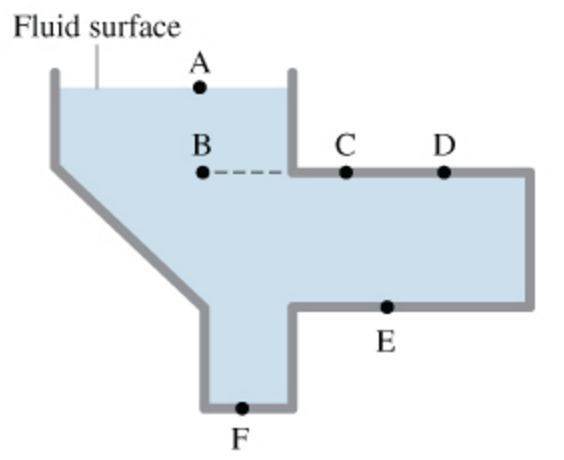# Problem: Rank from smallest to largest the pressures in the tank of motionless fluid shown in the figure

###### FREE Expert Solution

Pressure in liquids:

$\overline{){\mathbf{P}}{\mathbf{=}}{\mathbit{h}}{\mathbit{\rho }}{\mathbit{g}}}$

Pressure is directly proportional to the height from the surface of a liquid.###### Problem DetailsRank from smallest to largest the pressures in the tank of motionless fluid shown in the figure